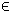## 7NLP Problem

TOMLAB requires that general nonlinear problems are defined in Matlab m-files. The function to be optimized must always be supplied. It is recommended that the user supply as many analytical functions as possible. There are six methods available for numerical differentiation and also two for automatic.

The constrained nonlinear programming problem is defined as:

 min x
f(x)

s/t
 xL ≤ x ≤ xU bL ≤ A x ≤ bU cL ≤ c(x) ≤ cU
(8)
where x, xL, xURn, f(x)R, ARm1 × n, bL,bURm1 and cL,c(x),cURm2.

Example problem:

 min x
f(x)=α ( x2x12)2+(1−x1 )2

s/t
 −10 ≤ x1 ≤ 2 −10 ≤ x2 ≤ 2 α=100
(9)

The following files define the problem in TOMLAB.

File: tomlab/quickguide/rbb_f.m, rbb_g.m, rbb_H.m, rbb_c.m, rbb_dc.m, rbb_d2c.m
```  f:   Function value
H:   Hessian matrix
c:   Nonlinear constraint vector
d2c: The second part of the Hessian to the Lagrangian function for the nonlinear constraints.
```
The following file illustrates how to solve this NLP (CON) problem in TOMLAB. Also view the m-files specified above for more information.

File: tomlab/quickguide/nlpQG.m

Open the file for viewing, and execute nlpQG in Matlab.
```% nlpQG is a small example problem for defining and solving
% nonlinear programming problems using the TOMLAB format.

Name = 'RBB Problem';
x_0 = [-1.2 1]';     % Starting values for the optimization.
x_L = [-10;-10];     % Lower bounds for x.
x_U = [2;2];         % Upper bounds for x.
fLowBnd = 0;         % Lower bound on function.

c_L = -1000;         % Lower bound on nonlinear constraints.
c_U = 0;             % Upper bound on nonlinear constraints.

Prob = conAssign('rbbQG_f', 'rbbQG_g', 'rbbQG_H', [], x_L, x_U, Name, x_0,...
[], fLowBnd, [], [], [], 'rbbQG_c', 'rbbQG_dc', 'rbbQG_d2c', [], c_L, c_U);

Prob.Warning = 0;    % Turning off warnings.

Result = tomRun('ucSolve', Prob, 1);  % Ignores constraints.

% Result = tomRun('conopt', Prob, 1);
% Result = tomRun('snopt', Prob, 1);```